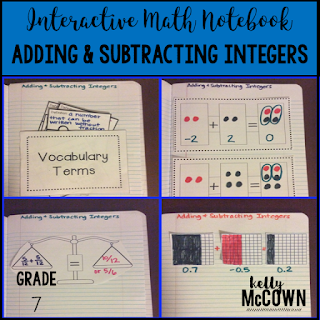## Sunday, September 3, 2017

### Teaching Integers with Interactive Math Notebooks

Are you getting ready to teach Integers?

There is a great resource for you to share with your students!These interactive notebook activities are intended to help students understand positive and negative numbers are used together to describe quantities having opposite values, understand rational numbers on a number line, and understand ordering and absolute value of rational numbers.

Included are:
-4 different Integer activities to engage students
-Teacher facilitated activity for 60-90 minutes of classroom time
-Activities include: Vocabulary, Practice of Integers, Ordering Integers, and Creating Number Lines with Integers
-Examples of Completed Interactive Math Notebook Activities are includedThese interactive notebook activities are intended to help students understand how to apply and extend previous understandings of addition and subtraction to rational numbers, describing situations in which opposite quantities combine, and showing that a number and its opposite have a sum of 0. Interpret sums of rational numbers by describing real world contexts. Students will also understand the additive inverse, apply properties of operations as strategies, and solve real-world problems with rational numbers.
Included are:
-4 different Integer activities to engage students
-Teacher facilitated activity for 60-90 minutes of classroom time
-Activities include: Vocabulary, Adding & Subtracting with Integer Chips, Adding and Subtracting Positive and Negative Fractions with a Balanced Scale, and Adding and Subtracting Positive and Negative Decimals
-Examples of Completed Interactive Math Notebook Activities are included

These interactive notebook activities are intended to help students understand how to apply and extend previous understandings of multiplication and division of fractions. Solve real-world and mathematical problems with four operations with rational numbers.

Included are:
-4 different Integer activities to engage students
-Teacher facilitated activity for 60-90 minutes of classroom time
-Activities include: Vocabulary, Multiplying & Dividing with Negative Integers, Multiplying & Dividing Negative and Positive Fractions with KCF Method, and Multiplying & Dividing Negative and Positive Decimals.
-Examples of Completed Interactive Math Notebook Activities are included

I hope these are great teaching resources for your classroom too. Engaging students in actively learning and practicing integers will ensure that they will master the concepts! Remember to keep it fun, interactive, and student centered.

Happy Teaching!